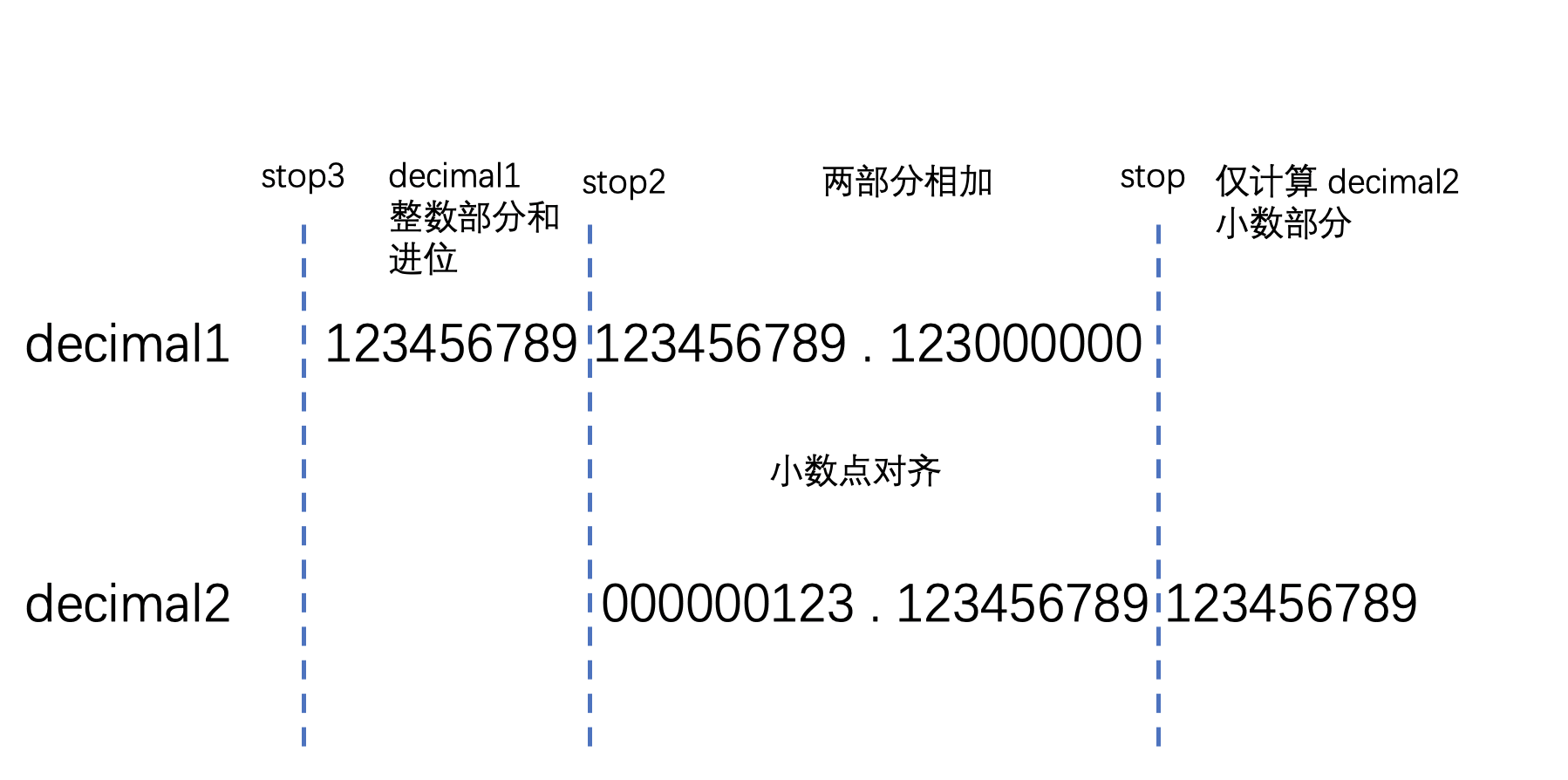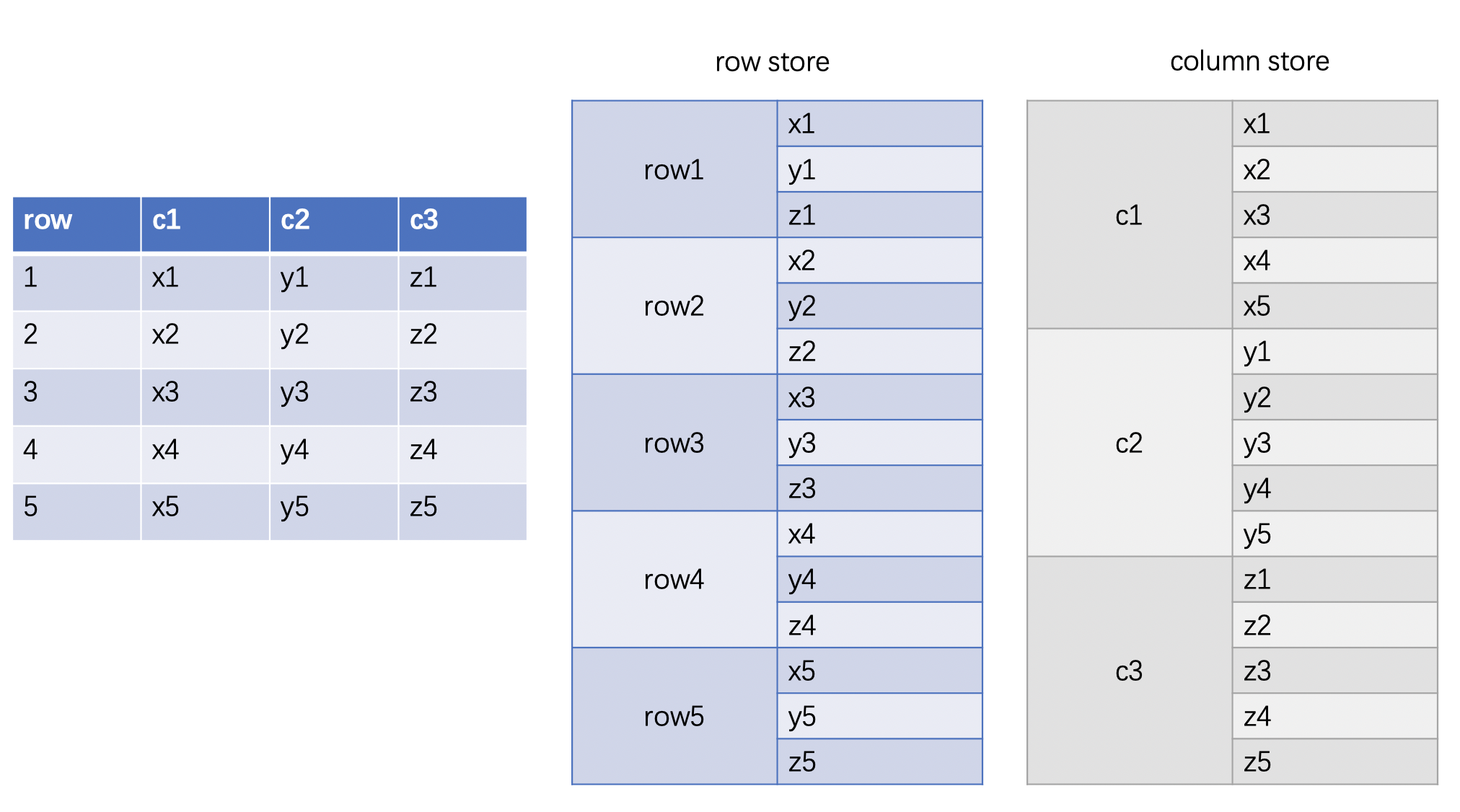PolarDB 数据库内核月报

# PolarDB 数据库内核月报 － 2021 / 03

## 创建和使用 decimal

decimal 的使用在多数数据库上都差不多, 下面以 MySQL 的 decimal 为例, 介绍 decimal 的基本使用方法.

### 在建表时定义 decimal

``````create table t(d decimal(5, 2));
``````

### 写入 decimal 数据

``````insert into t values(123.45);
insert into t values(123.4);
``````

``````+--------+
| d      |
+--------+
| 123.45 |
| 123.40 |
+--------+
``````

``````insert into t values(1123.45);
ERROR 1264 (22003): Out of range value for column 'd' at row 1
insert into t values(123.456);
Query OK, 1 row affected, 1 warning
show warnings;
+-------+------+----------------------------------------+
| Level | Code | Message                                |
+-------+------+----------------------------------------+
| Note  | 1265 | Data truncated for column 'd' at row 1 |
+-------+------+----------------------------------------+
select * from t;
+--------+
| d      |
+--------+
| 123.46 |
+--------+
``````

### 取出 decimal 进行计算

``````select d + 9999.999 from t;
+--------------+
| d + 9999.999 |
+--------------+
|    10123.459 |
+--------------+
``````

1. 加法/减法/sum：取两边最大的 scale
2. 乘法：两边的 scale 相加
3. 除法：被除数的 scale + div_precision_increment(取决于数据库实现)

## decimal 实现

1. 支持多大的 precision 和 scale
2. 在哪里存储 scale
3. 在连续乘法或除法时, scale 不断增长, 整数部分也不断扩大, 而存储的 buffer 大小总是又上限的, 此时应该如何处理？
4. 除法可能产生无限小数, 如何决定除法结果的 scale?
5. decimal 的表示范围和计算性能是否有冲突, 是否可以兼顾

### MySQL

``````typedef int32 decimal_digit_t;

struct decimal_t {
int intg, frac, len;
bool sign;
decimal_digit_t *buf;
};
``````

MySQL 的 decimal 使用一个长度为 len 的 decimal_digit_t (int32) 的数组 buf 来存储 decimal 的数字, 每个 decimal_digit_t 最多存储 9 个数字, 用 intg 表示整数部分的数字个数, frac 表示小数部分的数字个数, sign 表示符号. 小数部分和整数部分需要分开存储, 不能混合在一个 decimal_digit_t 中, 两部分都向小数点对齐, 这是因为整数和小数通常需要分开计算, 所以这样的格式可以更容易地将不同 decimal_t 小数和整数分别对齐, 便于加减法运算. len 在 MySQL 实现中恒为 9, 它表示存储的上限, 而 buf 实际有效的部分, 则是由 intg 和 frac 共同决定. 例如：

``````// 123.45 decimal(5, 2) 整数部分为 3,  小数部分为 2
decimal_t dec_123_45 = {
int intg = 3;
int frac = 2;
int len = 9;
bool sign = false;
decimal_digit_t *buf = {123, 450000000, ...};
};
``````

MySQL 需要使用两个 decimal_digit_t (int32) 来存储 123.45, 其中第一个为 123, 结合 intg=3, 它就表示整数部分为 123, 第二个数字为 450000000 (共 9 个数字), 由于 frac=2, 它表示小数部分为 .45

``````// decimal(81, 18) 63 个整数数字, 18 个小数数字, 用满整个 buffer
// 123456789012345678901234567890123456789012345678901234567890123.012345678901234567
decimal_t dec_81_digit = {
int intg = 63;
int frac = 18;
int len = 9;
bool sign = false;
buf = {123456789, 12345678, 901234567, 890123456, 789012345, 678901234, 567890123, 12345678, 901234567}
};
``````

• 准备步骤

在真正计算前, 还需要做一些准备工作：

1. MySQL 会将数字的个数 ROUND_UP 到 9 的整数倍, 这样后面就可以按照 decimal_digit_t 为单位来进行计算
2. 此外还要针对参与运算的两个 decimal 的具体情况, 计算结果的 precision 和 scale, 如果发现结果的 precision 超过了支持的上限, 那么会按照 decimal_digit_t 为单位减少小数的数字.
3. 在乘法过程中, 如果发生了 2 中的减少行为, 则需要 TRUNCATE 两个运算数, 避免中间结果超出范围.
• 加法主要步骤

首先, 因为两个数字的 precision 和 scale 可能不相同, 需要做一些准备工作, 将小数点对齐, 然后开始计算, 从最末尾小数开始向高位加, 分为三个步骤：

1. 将小数较多的 decimal 多出的小数数字复制到结果中
2. 将两个 decimal 公共的部分相加
3. 将整数较多的 decimal 多出的整数数字与进位相加到结果中

代码中使用了 stop, stop2 来标记小数点对齐后, 长度不同的数字出现差异的位置.

``````/* part 1 - max(frac) ... min (frac) */
while (buf1 > stop) *--buf0 = *--buf1;

/* part 2 - min(frac) ... min(intg) */
carry = 0;
while (buf1 > stop2) {
}

/* part 3 - min(intg) ... max(intg) */
buf1 = intg1 > intg2 ? ((stop3 = from1->buf) + intg1 - intg2)
: ((stop3 = from2->buf) + intg2 - intg1);
while (buf1 > stop3) {
}
``````• 乘法主要步骤

乘法引入了一个新的 dec2, 表示一个 64 bit 的数字, 这是因为两个 decimal_digit_t(int32) 相乘后得到的可能会是一个 64 bit 的数字. 在计算时一定要先把类型转换到 dec2(int64), 再计算, 否则会得到溢出后的错误结果.

``````typedef decimal_digit_t dec1;
typedef longlong dec2;
``````

乘法与加法不同, 乘法不需要对齐, 例如计算 11.11 * 5.0, 那么只要计算 1111*50=55550, 再移动小数点位置就能得到正确结果 55.550

MySQL 实现了一个双重循环将 decimal1 的 每一个 decimal_digit_t 与 decimal2 的每一个 decimal_digit_t 相乘, 得到一个 64 位的 dec2, 其低 32 位是当前的结果, 其高 32 位是进位.

``````for (buf1 += frac1 - 1; buf1 >= stop1; buf1--, start0--) {
carry = 0;
for (buf0 = start0, buf2 = start2; buf2 >= stop2; buf2--, buf0--) {
dec1 hi, lo;
dec2 p = ((dec2)*buf1) * ((dec2)*buf2);
hi = (dec1)(p / DIG_BASE);
lo = (dec1)(p - ((dec2)hi) * DIG_BASE);
carry += hi;
}
if (carry) {
if (buf0 < to->buf) return E_DEC_OVERFLOW;
}
for (buf0--; carry; buf0--) {
if (buf0 < to->buf) return E_DEC_OVERFLOW;
}
}
``````
• 除法主要步骤

除法使用的是 Knuth’s Algorithm D, 其基本思路和手动除法也比较类似.

首先使用除数的前两个 decimal_digit_t 组成一个试商因数, 这里使用了一个 norm_factor 来保证数字在不溢出的情况下尽可能扩大, 这是因为 decimal 为了保证精度必须使用整形来进行计算, 数字越大, 得到的结果就越准确.

``````norm2 = (dec1)(norm_factor * start2);
if (likely(len2 > 0)) norm2 += (dec1)(norm_factor * start2 / DIG_BASE);
``````

D3: 猜商, 就是用被除数的前两个 decimal_digit_t 除以试商因数

``````x = start1 + ((dec2)dcarry) * DIG_BASE;
y = start1;
guess = (norm_factor * x + norm_factor * y / DIG_BASE) / norm2;
``````

这里如果不乘 norm_factor, 则 start1 和 start2 都不会体现在结果之中.

D4: 将 guess 与除数相乘, 再从被除数中剪掉结果

``````for (carry = 0; buf2 > start2; buf1--) {
dec1 hi, lo;
x = guess * (*--buf2);
hi = (dec1)(x / DIG_BASE);
lo = (dec1)(x - ((dec2)hi) * DIG_BASE);
SUB2(*buf1, *buf1, lo, carry);
carry += hi;
}
carry = dcarry < carry;
``````

然后做一些修正, 移动向下一个 decimal_digit_t, 重复这个过程.

想更详细地了解这个算法可以参考 https://skanthak.homepage.t-online.de/division.html

### ClickHouse

ClickHouse 是列存, 相同列的数据会放在一起, 因此计算时通常也将一列的数据合成 batch 一起计算.``````class PODArray {
char * c_start          = null;
char * c_end            = null;
char * c_end_of_storage = null;
}
``````

``````template <typename T>
struct Decimal
{
using NativeType = T;
// ...
T value;
};
using Int32 = int32_t;
using Int64 = int64_t;
using Int128 = __int128;
using Decimal32 = Decimal<Int32>;
using Decimal64 = Decimal<Int64>;
using Decimal128 = Decimal<Int128>;
``````

``````ResultDataType type = decimalResultType(left, right, is_multiply, is_division);

int scale_a = type.scaleFactorFor(left, is_multiply);
int scale_b = type.scaleFactorFor(right, is_multiply || is_division);
OpImpl::vector_vector(col_left->getData(), col_right->getData(), vec_res,
scale_a, scale_b, check_decimal_overflow);
``````

• 加法主要步骤

ClickHouse 实现加法同样要先对齐, 对齐的方法是将 scale 较小的数字乘上一个系数, 使两边的 scale 相等.

``````bool overflow = false;
if constexpr (scale_left)
overflow |= common::mulOverflow(a, scale, a);
else
overflow |= common::mulOverflow(b, scale, b);

overflow |= Op::template apply<NativeResultType>(a, b, res);
``````

然后直接做加法即可. ClickHouse 在计算中也根据 decimal 的 precision 进行了细分, 对于长度没那么长的 decimal, 直接用 int32, int64 等原生类型计算就可以了, 这样大大提升了速度.

``````template <typename T>
inline bool addOverflow(T x, T y, T & res)
{
}

template <>
inline bool addOverflow(__int128 x, __int128 y, __int128 & res)
{
static constexpr __int128 min_int128 = __int128(0x8000000000000000ll) << 64;
static constexpr __int128 max_int128 = (__int128(0x7fffffffffffffffll) << 64) + 0xffffffffffffffffll;
res = x + y;
return (y > 0 && x > max_int128 - y) || (y < 0 && x < min_int128 - y);
}
``````
• 乘法主要步骤

同 MySQL, 乘法不需要对齐, 直接按整数相乘就可以了, 比较短的 decimal 同样可以使用 int32, int64 原生类型. int128 在溢出检测时被转换成 unsigned int128 避免溢出时的未定义行为.

``````template <typename T>
inline bool mulOverflow(T x, T y, T & res)
{
return __builtin_mul_overflow(x, y, &res);
}

template <>
inline bool mulOverflow(__int128 x, __int128 y, __int128 & res)
{
res = static_cast<unsigned __int128>(x) * static_cast<unsigned __int128>(y);    /// Avoid signed integer overflow.
if (!x || !y)
return false;

unsigned __int128 a = (x > 0) ? x : -x;
unsigned __int128 b = (y > 0) ? y : -y;
return (a * b) / b != a;
}
``````
• 除法主要步骤

先转换 scale 再直接做整数除法. 本身来讲除法和乘法一样是不需要对齐小数点的, 但是除法不一样的地方在于可能会产生无限小数, 所以一般数据库都会给结果一个固定的小数位数, ClickHouse 选择的小数位数是和被除数一样, 因此需要将 a 乘上 scale, 然后在除法运算的过程中, 这个 scale 被自然减去, 得到结果的小数位数就可以保持和被除数一样.

``````bool overflow = false;
if constexpr (!IsDecimalNumber<A>)
overflow |= common::mulOverflow(scale, scale, scale);
overflow |= common::mulOverflow(a, scale, a);
if (overflow)
throw Exception("Decimal math overflow", ErrorCodes::DECIMAL_OVERFLOW);

return Op::template apply<NativeResultType>(a, b);
``````

### 总结

MySQL 通过一个 int32 的数组来表示一个大数, ClickHouse 则是尽可能使用原生类型, GCC 和 Clang 都支持 int128 扩展, 这使得 ClickHouse 的这种做法可以比较方便地实现. MySQL 与 ClickHouse 的实现差别还是比较大的, 针对我们开始提到的问题, 分别来看看他们的解答.

1. precision 和 scale 范围, MySQL 最高可定义 precision=65, scale=30, 中间结果最多包含 81 个数字, ClickHouse 最高可定义 precision=38, scale=37, 中间结果最大为 int128 的最大值 -2^127 ~ 2^127-1.
2. 在哪里存储 scale, MySQL 是行式存储, 使用火山模型逐行迭代, 计算也是按行进行, 每个 decimal 都有自己的 scale；ClickHouse 是列式存储, 计算按列批量进行, 每行按照相同的 scale 处理能提升性能, 因此 scale 来自表达式解析过程中推导出来类型中.
3. scale 增长, scale 增长超过极限时, MySQL 会通过动态挤占小数空间, truncate 运算数, 尽可能保证计算完成, ClickHouse 会直接报溢出错.
4. 除法 scale, MySQL 通过 div_prec_increment 来控制除法结果的 scale, ClickHouse 固定使用被除数的 scale.
5. 性能, MySQL 使用了更宽的 decimal 表示, 同时要进行 ROUND_UP, 小数挤占, TRUNCATE 等动作, 性能较差, ClickHouse 使用原生的数据类型和计算最大限度地提升了性能.

## MySQL decimal

1. 乘法的 scale 会截断到 31, 且该截断是通过截断运算数字的方式来实现的, 例如: select 10000000000000000000000000000000.100000000 * 10000000000000000000000000000000 = 10000000000000000000000000000000.100000000000000000000000000000 * 10000000000000000000000000000000.555555555555555555555555555555 返回 1, 第二个运算数中的 .555555555555555555555555555555 全部被截断
2. MySQL 使用的 buffer 包含了 81 个 digit 的容量, 但是由于小数部分必须和整数部分分开, 因此很多时候无法用满 81 个 digit, 例如: select 99999999999999999999999999999999999999999999999999999999999999999999999999.999999 = 99999999999999999999999999999999999999999999999999999999999999999999999999.9 返回 1
3. 计算过程中如果发现整数部分太大会动态地挤占小数部分, 例如: select 999999999999999999999999999999999999999999999999999999999999999999999999.999999999 + 999999999999999999999999999999999999999999999999999999999999999999999999.999999999 = 999999999999999999999999999999999999999999999999999999999999999999999999 + 999999999999999999999999999999999999999999999999999999999999999999999999 返回 1
4. 除法计算中间结果不受 scale = 31 的限制, 除法中间结果的 scale 一定是 9 的整数倍, 不能按照最终结果来推测除法作为中间结果的精度, 例如 select 2.0000 / 3 * 3 返回 2.00000000, 而 select 2.00000 / 3 * 3 返回 1.999999998, 可见前者除法的中间结果其实保留了更多的精度.
5. 除法, avg 计算最终结果的小数部分如果正好是 9 的倍数, 则不会四舍五入, 例如: select 2.00000 / 3 返回 0.666666666, select 2.0000 / 3 返回 0.66666667